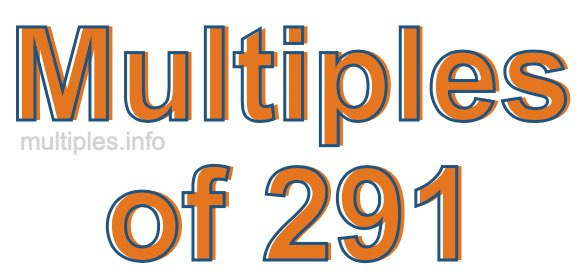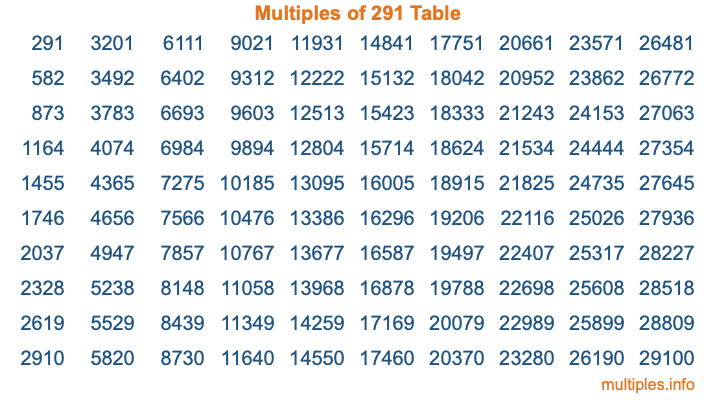Multiples of 291Welcome to the Multiples of 291 page. Here we will first teach you everything you will ever need to know about the multiples of 291, and then give you a study guide summary of everything we taught you to make sure you remember it all. Use this page to look up facts and learn information about the multiples of 291. This page will make you a multiples of two hundred ninety-one expert!

Definition of Multiples of 291
Multiples of 291 are all the numbers that when divided by 291 equal an integer. Each of the multiples of 291 are called a multiple. A multiple of 291 is created by multiplying 291 by an integer.

Therefore, to create a list of multiples of 291, you start with 1 multiplied by 291, then 2 multiplied by 291, then 3 multiplied by 291, and so on for as long as you want. Thus, the list of the first five multiples of 291 is 291, 582, 873, 1164, and 1455. To see a larger list of multiples of 291, see the printable image of Multiples of 291 further down on this page. We also have a category where you can choose any nth multiple of 291.

Multiples of 291 Checker
The Multiples of 291 Checker below checks to see if any number of your choice is a multiple of 291. In other words, it checks to see if there is any number (integer) that when multiplied by 291 will equal your number. To do that, we divide your number by 291. If the the quotient is an integer, then your number is a multiple of 291.

Is  a multiple of 291?

Least Common Multiple of 291 and ...
A Least Common Multiple (LCM) is the lowest multiple that two or more numbers have in common. This is also called the smallest common multiple or lowest common multiple and is useful to know when you are adding our subtracting fractions. Enter one or more numbers below (291 is already entered) to find the LCM.

Check out our LCM Calculator if you need more details about the Least Common Multiple or if you need the LCM for different numbers for adding and subtraction fractions.

nth Multiple of 291
As we stated above, 291 is the first multiple of 291, 582 is the second multiple of 291, 873 is the third multiple of 291, and so on. Enter a number below to find the nth multiple of 291.

th multiple of 291

Multiples of 291 vs Factors of 291
291 is a multiple of 291 and a factor of 291, but that is where the similarities end. All postive multiples of 291 are 291 or greater than 291. All positive factors of 291 are 291 or less than 291.

Below is the beginning list of multiples of 291 and the factors of 291 so you can compare:

Multiples of 291: 291, 582, 873, 1164, 1455, etc.

Factors of 291: 1, 3, 97, 291

As you can see, the multiples of 291 are all the numbers that you can divide by 291 to get a whole number. The factors of 291, on the other hand, are all the whole numbers that you can multiply by another whole number to get 291.

It's also interesting to note that if a number (x) is a factor of 291, then 291 will also be a multiple of that number (x).

Multiples of 291 vs Divisors of 291
The divisors of 291 are all the integers that 291 can be divided by evenly. Below is a list of the divisors of 291.

Divisors of 291: 1, 3, 97, 291

The interesting thing to note here is that if you take any multiple of 291 and divide it by a divisor of 291, you will see that the quotient is an integer.

Multiples of 291 Table
Below is an image of the first 100 multiples of 291 in a table. The table is in chronological order, column by column. The first column has the first ten multiples of 291, the second column has the next ten multiples of 291, and so on.The Multiples of 291 Table is also referred to as the 291 Times Table or Times Table of 291. You are welcome to print out our table for your studies.

Negative Multiples of 291
Although not often discussed or needed in math, it is worth mentioning that you can make a list of negative multiples of 291 by multiplying 291 by -1, then by -2, then by -3, and so on, to get the following list of negative multiples of 291:

-291, -582, -873, -1164, -1455, etc.

Multiples of 291 Summary
Below is a summary of important Multiples of 291 facts that we have discussed on this page. To retain the knowledge on this page, we recommend that you read through the summary and explain to yourself or a study partner why they hold true.

There are an infinite number of multiples of 291.

A multiple of 291 divided by 291 will equal a whole number.

291 divided by a factor of 291 equals a divisor of 291.

The nth multiple of 291 is n times 291.

The largest factor of 291 is equal to the first positive multiple of 291.

291 is a multiple of every factor of 291.

291 is a multiple of 291.

A multiple of 291 divided by a divisor of 291 equals an integer.

291 divided by a divisor of 291 equals a factor of 291.

Any integer times 291 will equal a multiple of 291.

Multiples of a Number
Here you can get the multiples of another number, all with the same attention to detail as we did for multiples of 291 on this page.

Multiples of
Multiples of 292
Did you find our page about multiples of two hundred ninety-one educational? Do you want more knowledge? Check out the multiples of the next number on our list!